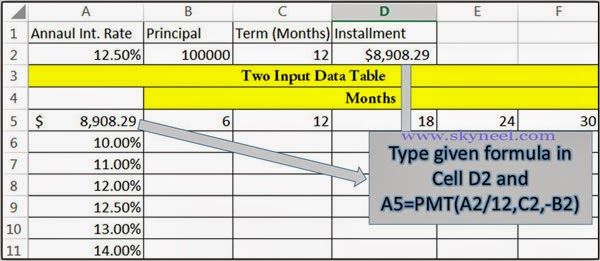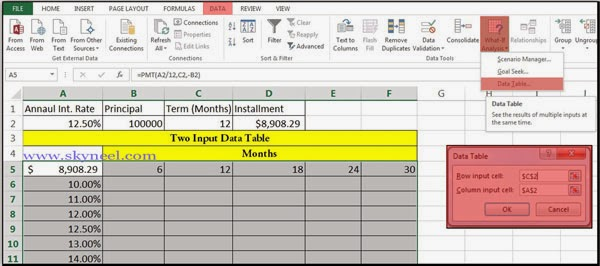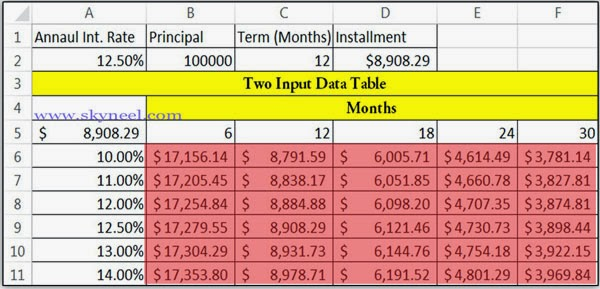# What If Analysis and Excel Two Input Data Table

What-IF-Analysis is an important part of Excel. You can create What If Analysis and Excel Two Input Data Table to modify 2 variables value.

What If Analysis and Excel Two Input Data Table permits you to change two variables value in your sheet and view how modifying the values for that variables affect the values on calculated by one or more formulas in active sheet.

## What If Analysis and Excel Two Input Data Table

What if analysis and excl two input data table allows you change the more than one variable values as per you need and get required result.

#### What-IF Analysis:

It is a method of modify the cell values and see how these modifications are taking affect the result of formulas on the worksheet. For example, varying the interest rate and term that is used in an amortization table to determine the amount of the payments.

#### Data Table:

Data table is an important part of the What-If-Analysis facility which can be used to change one or more than one variables and watch the result.

Formula: A series of numbers, reference of any cell, use of functions or any operators which is used in a cell that provide the final resulted value. In MS Excel, a formula always starts with an equal sign (=) operator. You can create two-input data tables from what if analysis facility in MS Excel, depending on the number of variables that you want to test. For Example: If you take loan from any bank on different interest rate and for different periods in months and you want to know how changing interest rate percentage variable affect the installment amount in active sheet, then you can use one input data table facility in MS Excel .

### How to create 2-Input Data Table

Step 1: If you want to create two input data table then first, you have to prepare following given sheet:Step 2: Type following given formula in both
Cells D2 & A5: =PMT(A2/12,C2,-B2)   then press enter key to view the result. Excel shows \$ 8908.29 as a result.Step 3: Select the range of cells from A5:F11, after that you have to click on the “Data” Tab. Now, click on the “What-If Analysis” option.  Click on the “Data Table” option and  type \$C\$2 in the Row Input Cell dialogue box & type \$A\$2 in Column Input Cell  box in Data Table dialog box. Now, click on the ok button.Step 4: Now, you will see the final result in two input data table: